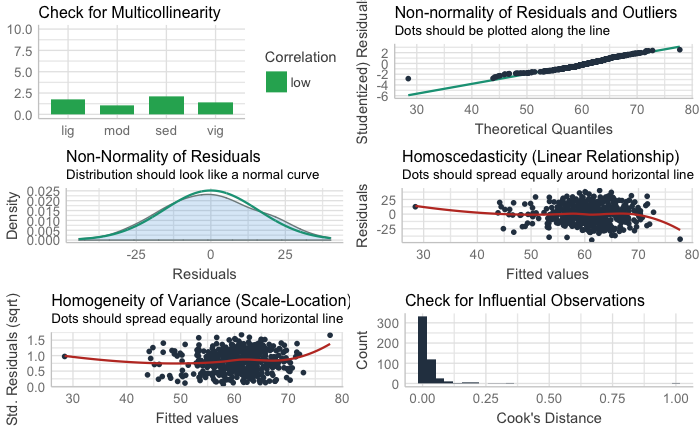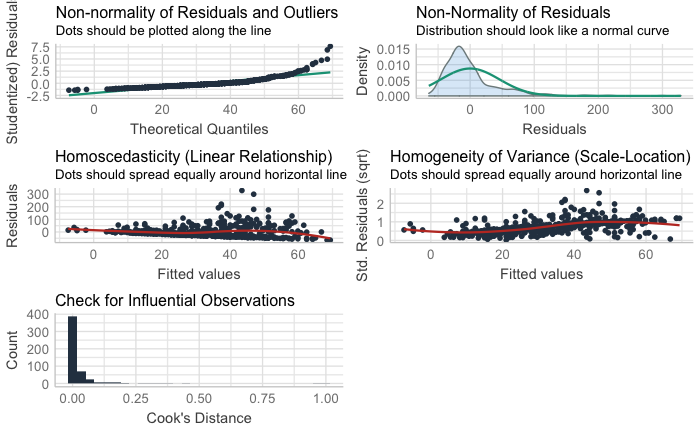This notebook contains the code and results of the data-analysis for the blog-post that I published here: https://hermandevries.nl/2020/09/23/relationships-between-hrv-sleep-and-physical-activity-in-personal-data/

I performed these analyses in RStudio 4.0.2 that uses R 1.3.1073.

# 0. Datamanagement

``````library(tidyverse) # Includes the packages dplyr and ggplot2 that I'll be using a lot.
library(here) # Useful for convenient file-management.
library(TTR) # Used to calculate the rolling averages with the SMA() function.

# Import the .csv file that I got from the Oura cloud dashboard:
``````Duplicated column names deduplicated: 'Sleep Timing' => 'Sleep Timing_1' Parsed with column specification:
cols(
.default = col_double(),
date = col_date(format = ""),
`Sleep Timing_1` = col_logical(),
`Bedtime Start` = col_datetime(format = ""),
`Bedtime End` = col_datetime(format = "")
)
See spec(...) for full column specifications.``````
``````# In my exported file, I had 2 days with missing nocturnal data. This were two nights with a very short sleep duration, which the Oura algorithm apparently filters out for some reason because it doesn't trust the observation - but I know these were actually correct. Luckily, the app itself still showed the data visually, so I added these two observations manually.

# My missing value on March 13, 2020:
df_oura\$`Total Sleep Time` = 121*60
df_oura\$`Total Bedtime` = 248*60
df_oura\$`Awake Time` = 127*60
df_oura\$`REM Sleep Time` = 3*60
df_oura\$`Light Sleep Time` = 52*60
df_oura\$`Deep Sleep Time` = 65*60
df_oura\$`Sleep Latency` = 43*60
df_oura\$`Sleep Score` = 38
df_oura\$`Sleep Efficiency` = 49
df_oura\$`Bedtime Start` = as.POSIXct("2020-03-12 22:03:00",
tz = "Europe/Amsterdam",
format = "%Y-%m-%d %H:%M:%OS")
df_oura\$`Bedtime End` = as.POSIXct("2020-03-13 02:11:00",
tz = "Europe/Amsterdam",
format = "%Y-%m-%d %H:%M:%OS")
df_oura\$`Average Resting Heart Rate` = 56
df_oura\$`Lowest Resting Heart Rate` = 52
df_oura\$`Average HRV` = 62
df_oura\$`Temperature Deviation (°C)` = 0.00
df_oura\$`Respiratory Rate` = 12.9

# Missing values for July 28th, 2020:
df_oura\$`Total Sleep Time` = 171*60
df_oura\$`Total Bedtime` = 191*60
df_oura\$`Awake Time` = 19*60
df_oura\$`REM Sleep Time` = 0*60
df_oura\$`Light Sleep Time` = 76*60
df_oura\$`Deep Sleep Time` = 95*60
df_oura\$`Sleep Latency` = 5*60
df_oura\$`Sleep Score` = 57
df_oura\$`Sleep Efficiency` = 90
df_oura\$`Bedtime Start` = as.POSIXct("2020-07-28 22:33:00",
tz = "Europe/Amsterdam",
format = "%Y-%m-%d %H:%M:%OS")
df_oura\$`Bedtime End` = as.POSIXct("2020-07-28 01:34:00",
tz = "Europe/Amsterdam",
format = "%Y-%m-%d %H:%M:%OS")
df_oura\$`Average Resting Heart Rate` = 43
df_oura\$`Lowest Resting Heart Rate` = 42
df_oura\$`Average HRV` = 92
df_oura\$`Temperature Deviation (°C)` = -0.10
df_oura\$`Respiratory Rate` = 12.4

# I like to rename some variables for more convenient coding, and will calculate some additional variables here that I'll use:
df_oura <- df_oura %>%
rename(
score_sleep = `Sleep Score`,
score_sleep_total = `Total Sleep Score`,
score_sleep_rem = `REM Sleep Score`,
score_sleep_deep = `Deep Sleep Score`,
score_sleep_efficiency = `Sleep Efficiency Score`,
score_sleep_restfullness = `Restfulness Score`,
score_sleep_latency = `Sleep Latency Score`,
score_sleep_timing = `Sleep Timing Score`,
tbt_min = `Total Bedtime`,
tst_min = `Total Sleep Time`,
awake_min = `Awake Time`,
rem_min = `REM Sleep Time`,
light_min = `Light Sleep Time`,
deep_min = `Deep Sleep Time`,
restless = `Restless Sleep`,
se = `Sleep Efficiency`,
sol_min = `Sleep Latency`,
timing = `Sleep Timing`,
start = `Bedtime Start`,
end = `Bedtime End`,
rhr_avg = `Average Resting Heart Rate`,
rhr_low = `Lowest Resting Heart Rate`,
hrv = `Average HRV`,
temp = `Temperature Deviation (°C)`,
rr = `Respiratory Rate`,
score_activity = `Activity Score`,
score_stay_active = `Stay Active Score`,
score_move_every_hour = `Move Every Hour Score`,
score_meet_daily_targets = `Meet Daily Targets Score`,
score_training_frequency = `Training Frequency Score`,
score_training_volume = `Training Volume Score`,
score_recovery_time = `Recovery Time Score`,
cal_act = `Activity Burn`,
cal_tot = `Total Burn`,
cal_tar = `Target Calories`,
steps = Steps,
active = `Daily Movement`,
sed = `Inactive Time`,
rest = `Rest Time`,
lig = `Low Activity Time`,
mod = `Medium Activity Time`,
vig = `High Activity Time`,
nwt = `Non-wear Time`,
met = `Average MET`,
inactive_long = `Long Periods of Inactivity`,
score_prev_night = `Previous Night Score`,
score_sleep_balance = `Sleep Balance Score`,
score_prev_activity = `Previous Day Activity Score`,
score_activity_balance = `Activity Balance Score`,
score_temp = `Temperature Score`,
score_rhr = `Resting Heart Rate Score`,
score_hrv_balance = `HRV Balance Score`,
score_recovery = `Recovery Index Score`
) %>%
mutate(
tbt_min = tbt_min / 60,
tbt_hr = tbt_min / 60,
tst_min = tst_min / 60,
tst_hr = tst_min / 60,
awake_min = awake_min / 60,
awake_hr = awake_min / 60,
rem_min = rem_min / 60,
rem_hr = rem_min / 60,
light_min = light_min / 60,
light_hr = light_min / 60,
deep_min = deep_min / 60,
deep_hr = deep_min / 60,
mvpa = mod+vig,
tst_hr_7dra = SMA(tst_hr, 7),
hrv_7dra = SMA(hrv, 7)
) %>%
dplyr::select(-`Sleep Timing_1`)

# Dump all the algorithmically determined scores, since I'm personally not interested in those:
df_oura <- df_oura %>% dplyr::select(-starts_with("score_"))

# Exploring the distribution of the HRV data:
hist_hrv <- ggplot(df_oura, aes(hrv)) +
geom_histogram() +
labs(
title = "Histogram of nocturnal HRV",
subtitle = "Measured with OURA ring (v2)",
x = "RMSSD (ms)",
y = "Count"
) +
theme_light()
hist_hrv

# That distribution actually looks relatively symmetric already. Let's logarithmically transform it just in case (this is a common practice in HRV research) and see if the distribution improves:
df_oura <- df_oura %>%
mutate(lnhrv = log(hrv))

# Create the actual histogram to see that distribution:
hist_lnhrv <- ggplot(df_oura, aes(lnhrv)) +
geom_histogram() +
labs(
title = "Histogram of natural log of nocturnal HRV",
subtitle = "Measured with OURA ring (v2)",
x = "lnRMSSD (ms)",
y = "Count"
) +
theme_light()
hist_lnhrv``````
``````
# That arguably looks slightly worse. Let's do some normality tests to see explore which of the two is the most normal (gaussian):
shapiro.test(df_oura\$hrv)``````
``````
Shapiro-Wilk normality test

data:  df_oura\$hrv
W = 0.99402, p-value = 0.04591``````
``shapiro.test(df_oura\$lnhrv)``
``````
Shapiro-Wilk normality test

data:  df_oura\$lnhrv
W = 0.95706, p-value = 6.638e-11``````
``````# Confirmed. The first is almost significant, the second very significant. I'll work with regular HRV in my analysis.

# Let's also check out the TST distribution:
hist_tst <- ggplot(df_oura, aes(tst_hr)) +
geom_histogram() +
labs(
title = "Histogram of TST",
subtitle = "Measured with OURA ring (v2)",
x = "TST (hr)",
y = "Count"
) +
theme_light()
hist_tst

shapiro.test(df_oura\$tst_hr)``````
``````
Shapiro-Wilk normality test

data:  df_oura\$tst_hr
W = 0.93266, p-value = 3.053e-14``````
``# Doesn't look normal (gaussian), but at least it looks reasonably symmetric so I'm not concerned with using it in the upcoming analyses.``

# 1. HRV versus physical activity

``library(performance) # A package with useful functions to easily assess the model diagnostics, e.g. with the check_model() function``
``````Warning messages:
1: Unknown or uninitialised column: `tst_hr_std`.
2: Unknown or uninitialised column: `tst_hr_7dra_std`.
3: Unknown or uninitialised column: `hrv_std`.
4: Unknown or uninitialised column: `hrv_7dra_std`. ``````
``````# First: See if time sedentary, light, moderate and vigorous physical activity predict the nocturnal HRV:
lm_hrv_pa <- lm(lead(hrv) ~ vig + mod + lig + sed, df_oura)
summary(lm_hrv_pa)``````
``````
Call:
lm(formula = lead(hrv) ~ vig + mod + lig + sed, data = df_oura)

Residuals:
Min      1Q  Median      3Q     Max
-44.295 -10.895  -0.433  11.107  40.240

Coefficients:
Estimate Std. Error t value Pr(>|t|)
(Intercept) 100.901963   8.723832  11.566  < 2e-16 ***
vig          -0.099211   0.018831  -5.268 2.06e-07 ***
mod          -0.198167   0.038302  -5.174 3.34e-07 ***
lig          -0.017947   0.012047  -1.490    0.137
sed          -0.049845   0.009714  -5.132 4.14e-07 ***
---
Signif. codes:  0 ‘***’ 0.001 ‘**’ 0.01 ‘*’ 0.05 ‘.’ 0.1 ‘ ’ 1

Residual standard error: 15.92 on 495 degrees of freedom
(1 observation deleted due to missingness)
Multiple R-squared:  0.1016,    Adjusted R-squared:  0.09432
F-statistic: 13.99 on 4 and 495 DF,  p-value: 7.998e-11``````
``check_model(lm_hrv_pa)````````# Yes they do, all of them except for light PA. Model diagnostics look great. 9.4% explained variance.

# Second: See if my nocturnal HRV predicts my subsequent daytime moderate-to-vigorous physical activity (MVPA).
lm_mvpa_hrv <- lm(mvpa ~ hrv, df_oura)
summary(lm_mvpa_hrv) # It does. 8.8% explained variance.``````
``````
Call:
lm(formula = mvpa ~ hrv, data = df_oura)

Residuals:
Min     1Q Median     3Q    Max
-64.48 -25.78 -11.29  10.03 328.55

Coefficients:
Estimate Std. Error t value Pr(>|t|)
(Intercept) -19.5537     7.6864  -2.544   0.0113 *
hrv           0.8631     0.1221   7.071 5.21e-12 ***
---
Signif. codes:  0 ‘***’ 0.001 ‘**’ 0.01 ‘*’ 0.05 ‘.’ 0.1 ‘ ’ 1

Residual standard error: 45.6 on 499 degrees of freedom
Multiple R-squared:  0.09108,   Adjusted R-squared:  0.08926
F-statistic:    50 on 1 and 499 DF,  p-value: 5.212e-12``````
``check_model(lm_mvpa_hrv)``
``Not enough model terms in the conditional part of the model to check for multicollinearity.````````# Model diagnostics look iffy. Redisuals are clearly not normally distributed, and the homoscedasticity graph shows a clear floor effect of the HRV, whereas the Q-Q plot shows that particularly for very high HRV values, the residuals move away from the line. Perhaps this same model but with the logarithmically transformed HRV is better?
lm_mvpa_lnhrv <- lm(mvpa ~ lnhrv, df_oura)
summary(lm_mvpa_lnhrv)``````
``````
Call:
lm(formula = mvpa ~ lnhrv, data = df_oura)

Residuals:
Min     1Q Median     3Q    Max
-54.40 -27.71 -12.69  10.49 328.84

Coefficients:
Estimate Std. Error t value Pr(>|t|)
(Intercept) -152.266     27.574  -5.522 5.40e-08 ***
lnhrv         45.549      6.766   6.732 4.61e-11 ***
---
Signif. codes:  0 ‘***’ 0.001 ‘**’ 0.01 ‘*’ 0.05 ‘.’ 0.1 ‘ ’ 1

Residual standard error: 45.8 on 499 degrees of freedom
Multiple R-squared:  0.08326,   Adjusted R-squared:  0.08143
F-statistic: 45.32 on 1 and 499 DF,  p-value: 4.605e-11``````
``check_model(lm_mvpa_lnhrv)``
``Not enough model terms in the conditional part of the model to check for multicollinearity.``